# Matrices: An introduction

This tutorial discusses some of the ins and outs of matrices. Matrices can be fun, but more importantly, they can really save you time. Author: Lionel Brits

Introduction

A matrix is, by definition, a rectangular array of numeric or algebraic quantities which are subject to mathematical operations. Matrices can be defined in terms of their dimensions (number of rows and columns). Let us take a look at a matrix with 4 rows and 3 columns (we denote it as a 4×3 matrix and call it A):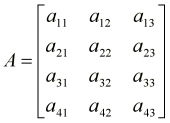Each individual item in a matrix is called a cell, and can be denoted by the particular row and column it resides in. For instance, in matrix A, element a32 can be found where the 3rd row and the 2nd column intersect.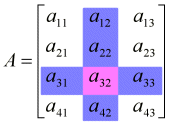What are they used for?

Matrices are used to represent complicated or time-consuming mathematical operations. A single matrix can hold an infinite number of calculations, which can then be applied to a number, vector, or another matrix. There are several operations that can be done on matrices, including addition, multiplication and inverse calculation; some of which will be discussed shortly. Operations done on one matrix can be transferred to another matrix simply by concatenating the two (by matrix multiplication). Matrices often find their use in 3 dimensional applications, were numerous identical operations are performed on thousands of vectors 30 or 40 times a second. Combining all these operations in one single matrix significantly improves the speed and functionality of a 3D rendering pipeline. Matrices are also used in financial processes (again, where a large number of data has to be processed in a similar fashion).

Operations on Matrices

Addition and subtraction operations can easily be performed on matrices, provided the matrices have the same dimensions. All that is required is to add or subtract the corresponding cells of each matrix involved in the operation. Let us take a look at the addition of two 2×3 matrices, A and B: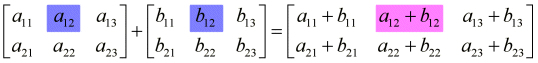2.Scalar Multiplication

Multiplying a matrix by a scalar value involves multiplying every element of the matrix by that value. Here we multiply our 2×3 matrix A by a scalar value β: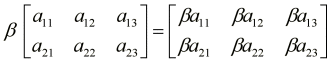3.Matrix Multiplication

The multiplication operation on matrices differs significantly from its real counterpart. One major difference is that multiplication can be performed on matrices with different dimensions. The first restriction is that the first matrix has to have the same amount of columns as the second has rows. The reason for this will become clear shortly. Another thing to note is that matrix multiplication is not commutative i.e., (CD) does not equal (DC).

The procedure for matrix multiplication is rather simple. First, we determine the dimensions of the resultant matrix. All we require is that there are as many columns in the first matrix as there are rows in the second. A simple way of determining is to look at the nearest and farthest dimensions of two matrix symbols written next to each other, for instance: C[2x3] D[3x2]. The nearest dimensions are both equal to 3, and so we know that the operation is possible. The farthest dimensions will give us the dimensions of the product matrix, so our result will be a 2×2 matrix. The general rule says that in order to perform the multiplication AB, where A is a (m x n) matrix and B a (k x l) matrix, we must have n=k. The result will be a (m x l) matrix.

Performing the operation product involves multiplying the cells of a particular rows in the first matrix by the cells of a particular column in the second matrix, adding the products, and storing the result in the cell of the resultant matrix whose coordinates correspond to the row of the first matrix and the column of the second matrix. For instance, in AB = C, if we want to find the value of c12, we must multiply the cells of row 1 in the first matrix by the cells of column 2 in the second matrix and sum the results.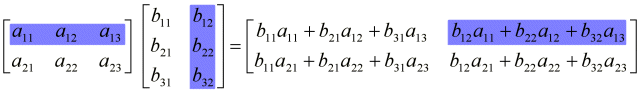4.Matrix/Vector Multiplication

We can also multiply matrices and vectors together, since a vector is nothing more than a 1-column matrix. Consider a 4×4 matrix M which comprises an arbitrary number of transformations (rotation, scaling, translation, etc.) which are to be applied to a 4×1 vector, S. The resultant 4×1 vector R obtained by multiplying M and S together is then a transformation of S.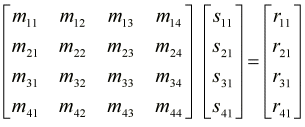5.Coding with matrices

How do we define a matrix in C++ you ask? Simple. If a matrix is a two dimensional array of numbers, by definition, then we should be able to define it in C++ that way too. Here’s how:

`typedef float MATRIX; // A 4×4 matrix`

The simplest thing you would want to do is concatenate (multiply) two matrices. We perform the operation, as mentioned earlier, like so:

```// matmult: Multiplies matrices A and B, storing the result in A void matmult(MATRIX &A, MATRIX B) {```
```MATRIX conc; // Multiplies by rows and columns for (int i = 0; i <4; i++) for (int j = 0; j < 4; j++) {```
```conc[i][j] = (A[j] * B[i])+ (A[j] * B[i])+ (A[j] * B[i])+ (A[j] * B[i]); }```
```// Copy result matrix into matrix A for (int p = 0; p < 4; p++) for (int q = 0; q < 4; q++) a[p][q] = conc[p][q]; }```

Note: The code references cell yx as cell xy. This may be changed in the near future for the sake of clarity.

You also need to initialize a matrix before you begin to work with it (unless you are simply copying from one matrix to another). Here’s the code to do so:

```// matinit: Initializes a matrix, and sets to 'identity' void matinit(MATRIX &a) {```
```for (int i = 0; i < 4; i++) for (int j = 0; j < 4; j++) a[i][j] = 0.0f; a = 1.0f;```
```a = 1.0f; a = 1.0f; a = 1.0f; }```

Note: The code references cell yx as cell xy. This may be changed in the near future for the sake of clarity.

Matrices are essentially useless unless you apply them to something. In 3D programming, you would want to apply them to vectors. By applying say, a rotation matrix, to a vector, we can rotate that vector around the origin in 3D space. 3D vectors are simply 3×1 matrices. Here is the code to do so:

```// vecmatmult: Multiplies vector V and matrix B void vecmatmult(VECTOR &v, MATRIX b) {```
```float x, y, z, w = 1; x = (v.x * b) + (v.y * b) + (v.z * b) + (w * b); y = (v.x * b) + (v.y * b) + (v.z * b) + (w * b); z = (v.x * b) + (v.y * b) + (v.z * b) + (w * b);```
```v.x = x; v.y = y; v.z = z; }```

Note: The code references cell yx as cell xy. This may be changed in the near future for the sake of clarity.

What is w you ask? The w is simply to accommodate the translation cells, since the vector is only 3×1 and the matrix is 4×4.

Useful matrices

Here are a couple of useful matrices that you can use. Most of them are for 3D geometry and are 4×4 matrices. I will add some more in the near future and also show how they are derived. Here they are:

The identity matrix is a matrix that does not affect the contents stored in another matrix when multiplied. It can be used as a basis for for constructing other matrices. An identity matrix can be any size, as long as it’s diagonal consists of 1’s and all other cells are filled with zeros, as shown: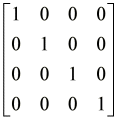The translation matrix is a matrix that can be used to translate vectors, such points, in n-dimensional space. Here, translations are for 3-dimensional space, where tx, ty and tz relate to displacements in the x, y and z dimensions respectively. Each component of the translation is added to it’s respective vector component. Notice how this matrix is based on the identity matrix: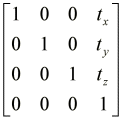The scaling matrix is a matrix that can be used to scale vectors, such points, in n-dimensional space. Here, scaling values are for 3-dimensional space, where sx, sy and sz relate to scaling factors in the x, y and z dimensions respectively. Notice, once again, how this matrix is based on the identity matrix: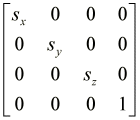The x-rotation matrix is a matrix that can be used to rotate vectors around the x-axis in 3-dimensional space. Theta (q) is the angle at which the vector is rotated. Lets look at how this matrix is derived:

The process of rotating a vector around the x-axis in 3D space is as follows:

x’ = x
y’ = (y*cosq) + (z*sinq)
z’ = (z*-sinq) - (y*cosq)

When applying this matrix to a vector (by means of a dot product), each of those transformations is only applied once, to the appropriate vector component. Again, these matrices are based on the identity matrix: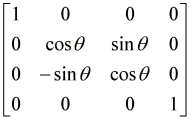The y-rotation matrix is a matrix that can be used to rotate vectors around the y-axis in 3-dimensional space. Theta (q) is the angle at which the vector is rotated. Lets look at how this matrix is derived:

Here, the process of rotation is similar to that of the x-rotation matrix:

x’ = (x*cosq) - (z*sinq)
y’ = y
z’ = (x*sinq) + (z*cosq)

Here is the matrix: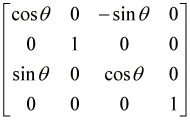The z-rotation matrix is a matrix that can be used to rotate vectors around the z-axis in 3-dimensional space. Again, theta (q) is the angle at which the vector is rotated. Lets look at a simple derivation:

Here, the process of rotation is similar to that of the x-rotation matrix:

x’ = (x*cosq) + (y*sinq)
y’ = -(x*sinq) + (y*cosq)
z’ = z

Here it is: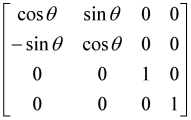### 3 Responses to “Matrices: An introduction”

1.www.cpp-home.com says:

Reposted it. Greetings from the Speedy DNS

2.zerodtkjoe says:

Thanks for the info

3.bet365 says:

how are you!This was a really wonderful post!
I come from milan, I was luck to look for your subject in bing
Also I get a lot in your website really thank your very much i will come every day